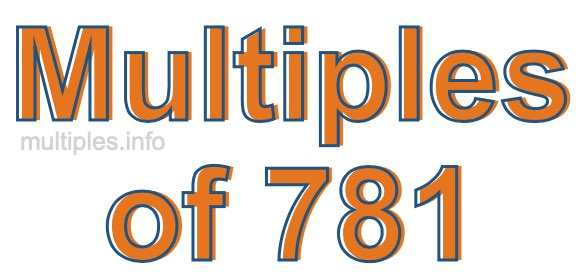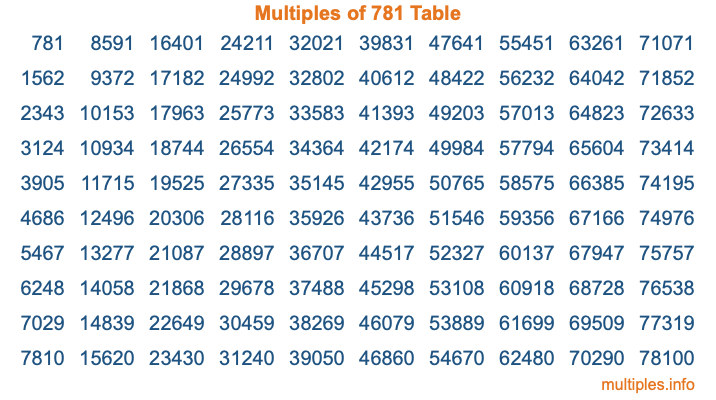Multiples of 781Welcome to the Multiples of 781 page. Here we will first teach you everything you will ever need to know about the multiples of 781, and then give you a study guide summary of everything we taught you to make sure you remember it all. Use this page to look up facts and learn information about the multiples of 781. This page will make you a multiples of seven hundred eighty-one expert!

Definition of Multiples of 781
Multiples of 781 are all the numbers that when divided by 781 equal an integer. Each of the multiples of 781 are called a multiple. A multiple of 781 is created by multiplying 781 by an integer.

Therefore, to create a list of multiples of 781, you start with 1 multiplied by 781, then 2 multiplied by 781, then 3 multiplied by 781, and so on for as long as you want. Thus, the list of the first five multiples of 781 is 781, 1562, 2343, 3124, and 3905. To see a larger list of multiples of 781, see the printable image of Multiples of 781 further down on this page. We also have a category where you can choose any nth multiple of 781.

Multiples of 781 Checker
The Multiples of 781 Checker below checks to see if any number of your choice is a multiple of 781. In other words, it checks to see if there is any number (integer) that when multiplied by 781 will equal your number. To do that, we divide your number by 781. If the the quotient is an integer, then your number is a multiple of 781.

Is  a multiple of 781?

Least Common Multiple of 781 and ...
A Least Common Multiple (LCM) is the lowest multiple that two or more numbers have in common. This is also called the smallest common multiple or lowest common multiple and is useful to know when you are adding our subtracting fractions. Enter one or more numbers below (781 is already entered) to find the LCM.

Check out our LCM Calculator if you need more details about the Least Common Multiple or if you need the LCM for different numbers for adding and subtraction fractions.

nth Multiple of 781
As we stated above, 781 is the first multiple of 781, 1562 is the second multiple of 781, 2343 is the third multiple of 781, and so on. Enter a number below to find the nth multiple of 781.

th multiple of 781

Multiples of 781 vs Factors of 781
781 is a multiple of 781 and a factor of 781, but that is where the similarities end. All postive multiples of 781 are 781 or greater than 781. All positive factors of 781 are 781 or less than 781.

Below is the beginning list of multiples of 781 and the factors of 781 so you can compare:

Multiples of 781: 781, 1562, 2343, 3124, 3905, etc.

Factors of 781: 1, 11, 71, 781

As you can see, the multiples of 781 are all the numbers that you can divide by 781 to get a whole number. The factors of 781, on the other hand, are all the whole numbers that you can multiply by another whole number to get 781.

It's also interesting to note that if a number (x) is a factor of 781, then 781 will also be a multiple of that number (x).

Multiples of 781 vs Divisors of 781
The divisors of 781 are all the integers that 781 can be divided by evenly. Below is a list of the divisors of 781.

Divisors of 781: 1, 11, 71, 781

The interesting thing to note here is that if you take any multiple of 781 and divide it by a divisor of 781, you will see that the quotient is an integer.

Multiples of 781 Table
Below is an image of the first 100 multiples of 781 in a table. The table is in chronological order, column by column. The first column has the first ten multiples of 781, the second column has the next ten multiples of 781, and so on.The Multiples of 781 Table is also referred to as the 781 Times Table or Times Table of 781. You are welcome to print out our table for your studies.

Negative Multiples of 781
Although not often discussed or needed in math, it is worth mentioning that you can make a list of negative multiples of 781 by multiplying 781 by -1, then by -2, then by -3, and so on, to get the following list of negative multiples of 781:

-781, -1562, -2343, -3124, -3905, etc.

Multiples of 781 Summary
Below is a summary of important Multiples of 781 facts that we have discussed on this page. To retain the knowledge on this page, we recommend that you read through the summary and explain to yourself or a study partner why they hold true.

There are an infinite number of multiples of 781.

A multiple of 781 divided by 781 will equal a whole number.

781 divided by a factor of 781 equals a divisor of 781.

The nth multiple of 781 is n times 781.

The largest factor of 781 is equal to the first positive multiple of 781.

781 is a multiple of every factor of 781.

781 is a multiple of 781.

A multiple of 781 divided by a divisor of 781 equals an integer.

781 divided by a divisor of 781 equals a factor of 781.

Any integer times 781 will equal a multiple of 781.

Multiples of a Number
Here you can get the multiples of another number, all with the same attention to detail as we did for multiples of 781 on this page.

Multiples of
Multiples of 782
Did you find our page about multiples of seven hundred eighty-one educational? Do you want more knowledge? Check out the multiples of the next number on our list!